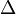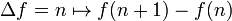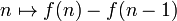# Discrete derivative

## Definition

The term discrete derivative is a loosely used term to describe an analogue of derivative for a function whose domain is discrete. The idea is typically to define this as a difference quotient rather than the usual continuous notion of derivative, which is defined as a limit of a difference quotient.

The typical case of interest is a function defined on the set of integers, or some contiguous subset of the set of integers (for instance, all integers from$a$ to$b$, where$a < b$ are integers). There are two related notions:

• The forward difference operator, sometimes denoted$\Delta$, is defined as follows for a function$f$:$\Delta f = n \mapsto f(n + 1) - f(n)$

This can be thought as a difference quotient between$n$ and$n + 1$. Note that it is analogous to the right hand derivative.

• The backward difference operator is defined as:$n \mapsto f(n) - f(n - 1)$

This can be thought as a difference quotient between$n$ and$n - 1$. Note that it is analogous to the left hand derivative.

In practice, we simply choose one of these as the notion of discrete derivative and stick with it. The reason is that the forward difference operator of$f$ at$n$ equals the backward difference operator of$f$ at$n + 1$, so we do not in fact lose any information by considering only one of these operators as the discrete derivative.